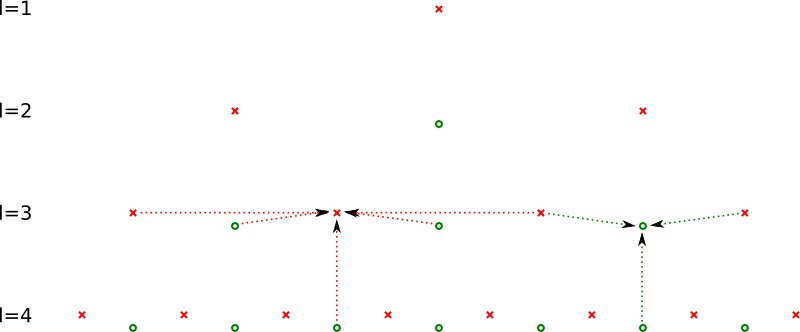SG++-Doxygen-Documentation
sgpp::base::ConvertPrewaveletToLinear Class Reference

Class that implements the transformation of a hierarchical prewavelet sparse grid to a hierarchical linear sparse grid. More...

#include <ConvertPrewaveletToLinear.hpp>

## Public Member Functions

ConvertPrewaveletToLinear (GridStorage &storage)
Constructor, must be bind to a grid. More...

void operator() (DataVector &source, DataVector &result, grid_iterator &index, size_t dim)
Converts a given prewavelet base to a normal linear base. More...

~ConvertPrewaveletToLinear ()
Destructor. More...

## Protected Types

typedef GridStorage::grid_iterator grid_iterator

typedef index_t index_type

typedef level_t level_type

## Protected Attributes

GridStoragestorage
the grid object More...

## Detailed Description

Class that implements the transformation of a hierarchical prewavelet sparse grid to a hierarchical linear sparse grid.

Therefore the ()operator is implemented in order to use the sweep algorithm for the grid traversal. Let the coefficients from the hat basis be $$h_{l,i}$$ and from the prewavelet basis $$u_{l,i}$$. To calculate the surplusses, temp values are needed:

$(l,i)\neq G_{n}^{1}:t_{l,i}=-\frac{6}{10}u_{l,i\pm1}+t_{l+1,2i}$

All temp values for levels greater than the maximal level of the grid are set to 0. The actual transformation is calculated as follows:

\begin{eqnarray*} h_{l,i}&=&u_{l,i}+\frac{1}{10}u_{l,i\pm2}+t_{l+1,2i}-\frac{1}{2}t_{l,i\pm1}\qquad\mbox{if \ensuremath{(l,i)} is an inner point}\\h_{l,i}&=&\frac{9}{10}u_{l,i}+\frac{1}{10}u_{l,i\pm2}+t_{l+1,2i}-\frac{1}{2}t_{l,i\pm1}\qquad\mbox{if \ensuremath{(l,i)} is at border} \end{eqnarray*}

The picture depicts all needed variables in oder to perform the transformation:This picture shows all involved gridpoints (red crosses) and temp values (green circles) to calculate the new hierarchical coefficients (red arrows) and new temp values (green arrows).

## ◆ grid_iterator

 protected

## ◆ index_type

 protected

## ◆ level_type

 protected

## ◆ ConvertPrewaveletToLinear()

 sgpp::base::ConvertPrewaveletToLinear::ConvertPrewaveletToLinear ( GridStorage & storage )
explicit

Constructor, must be bind to a grid.

Parameters
 storage the grid storage object of the the grid, on which the hierarchisation should be executed

## ◆ ~ConvertPrewaveletToLinear()

 sgpp::base::ConvertPrewaveletToLinear::~ConvertPrewaveletToLinear ( )

Destructor.

## ◆ operator()()

 void sgpp::base::ConvertPrewaveletToLinear::operator() ( DataVector & source, DataVector & result, grid_iterator & index, size_t dim )

Converts a given prewavelet base to a normal linear base.

## ◆ storage

 GridStorage& sgpp::base::ConvertPrewaveletToLinear::storage
protected

the grid object

Referenced by operator()().

The documentation for this class was generated from the following files: